# Graph regions

Regions are areas of a Minitab graph that can be resized, relocated, and formatted with color, fill patterns, edge line types, and more.

## About graph, figure, and data regions

Knowing where regions are is important for graph editing, especially if you have multiple graphs within a single graph region.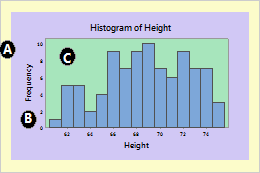In this illustration, the graph, figure, and data regions are labeled.
A: Graph region

The area just within the graph border that encompasses all the graphs, including their figure regions, data regions, legends, and tables. When you print a graph, the graph region corresponds to one sheet of paper.

Usually, the graph region contains a single graph. A layout may include several different graphs, but it still has only one graph region.

B: Figure region
The area just within the graph region that includes the data region, axes, labels, titles, and footnotes. Usually, graphs, including overlaid and paneled graphs, have only one figure region. You can only have multiple figure regions when you use the graph layout tool. A single figure region is transparent and takes up the entire graph region.
C: Data regions

The area in which the data are displayed. You can put additional text, lines, symbols, and polygons in the data region. Pie charts and 3D graphs do not have data regions.

## Modify or move a region

When you are editing a graph, the regions can be resized, relocated, and formatted with color, fill patterns, edge line types, and more. In addition, you can change the fonts for the legend and table regions. To edit a graph, double-click on the graph to open the Edit Graph dialog box. Then you can do one of the following:

• To modify a region, double-click it and complete the dialog box.
• To move a region, drag it to where you want it.
• To resize a region, click it and drag the sizing handles.

Each region uses a coordinate system for positioning items within it. You might use a region's coordinate system if you are writing a macro, but usually, it is easier to position regions and annotations by dragging them.

Data Units
Use data units to position items such as:
• Annotation, including text, lines, markers, and polygons
• Scale elements, including tick marks and reference lines

Data regions use the graph scales as coordinate systems. A categorical scale uses index values incrementing from left to right. The index value 1 signifies the first category, 2 signifies the second, and so on. A value of 1.5 corresponds to the point halfway between the first and second category.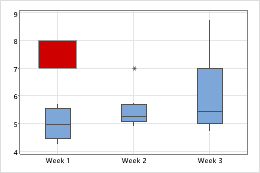Coordinates on a graph with a categorical scaleThe red rectangle is at Y minimum = 7, Y maximum = 8, X minimum = 0.75, and X maximum = 1.25.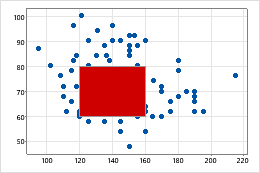Coordinates on a graph with a continuous scaleThe red rectangle is at Y minimum = 60, Y maximum = 80, X minimum = 120, X maximum = 160.
Figure Units
Use figure units to position the following items that are specific to a particular graph:
• The data, legend, and output table regions
• Annotation items such as titles and footnotes
• Scale elements such as axis labels and tick labels

The figure coordinate system ranges from 0 to 1 in each direction, with x beginning at the left edge of the region and y beginning at the bottom.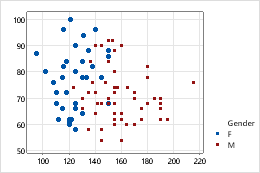Legend positioned by figure unitsThe legend region is at coordinates X minimum = 0.8038, X maximum = 0.9923, Y minimum = 0.1264, Y maximum = 0.3448.
Graph Units
Use graph units to position items relative to the entire graph. For example, position items such as:
• One or more figure regions on a graph region
• Annotation items for the graph region

The graph region coordinate system ranges from 0 to 1 in each direction, with the initial value of x at the left edge of the graph region and the initial value of y at the bottom.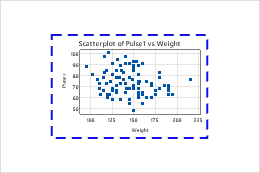Figure region placed by graph units The figure region is bounded by a dotted line and positioned at graph coordinates X minimum = 0.2, X maximum = 0.8, Y minimum = 0.2, Y maximum = 0.8.

## Specify the position of a graph region or annotation using coordinates

Each region uses a coordinate system for positioning items within it. You might use a region's coordinate system if you are writing a macro, but usually it is easier to position regions and annotations by dragging them.

###### Tip

If you add annotation or move regions on a graph, you can copy the coordinates for those items. With the graph in editing mode, click the Copy Command Language button on the toolbar. The coordinates are copied, along with the remainder of the command language that you can use to recreate the graph.

1. Double-click the graph.
2. Double-click the region or annotation item on the graph to open the editing dialog box.
3. Click the Location tab and choose Custom.
4. Enter the coordinates.
5. Click OK.

## Change the attributes of a graph, figure, or data region

To change the attributes of a region, use the following steps:
1. Double-click the graph.
2. Double-click the scale to open the Edit Data Region or Edit Graph and Figure Regions dialog box.
3. On the Graph Attributes tab (for graph and figure regions), or the Attributes tab (for data regions), change the fill pattern, border lines, and fill lines.
For example, the following individual vale plot has a customized graph region and data region. The following 3D surface plot has a customized graph region.
###### Note

The figure region, which is in front of the graph region and behind the data region, is transparent by default.

By using this site you agree to the use of cookies for analytics and personalized content.  Read our policy